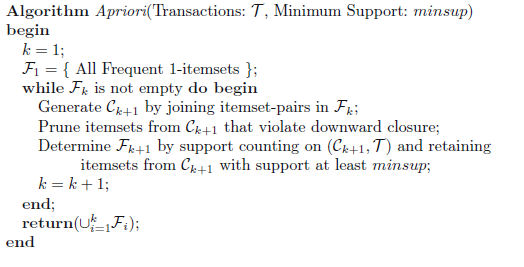# Association Rule Mining

Association rule mining is the basis for pattern recognition within multiple applications to find frequent relations, causal structures or correlations between occurrences of data. Common applications of association rule mining are market basket analysis, fraud detection, medical diagnosis, cross-marketing etc. Association guides decision making based on the historical data that exists.

An association rule is represented in the format:

• Antecedent -> Consequent,

which are defined by the measures of interestingness i.e support and confidence following a given threshold. These established rules can be be categorised as: actionable rules- having relevant insights, trivial rules- known to people with domain knowledge and inexplicable- relationships have no explainable insight and impact. There a couple of measures used with regard to the association rules.

### The measures of interestingness

Support is the frequency of the rule within the data. It is given by:

• support(A -> B) = p(A and B)

Confidence is the percentage of occurrences containing A which also contain B. Given by:

• confidence(A -> B) = p(B|A) = sup(A,B)/sup(A)

### Apriori algorithm

This algorithm relates to associative rule mining from frequent occurrence sets by relying on the downward closure property.

“The Apriori algorithm generates candidates with smaller length k first and counts their supports before generating candidates of length $$(k+1)$$. The resulting frequent k-itemsets are used to restrict the number of $$(k + 1)$$-candidates with the downward closure property”

This phenomenon restricts the cost for generating frequent patterns or occurrences based on the support threshold defined.There are other frequent itemset mining algorithms like enumeration tree, recursive growth suffix based pattern growth

### Anti monotone property

To implement the anti monotone property while generating the association rules; the following theorem is held on the confidence measure;

“Let $$Y$$ be an itemset and $$X$$ is a subset of $$Y$$ . If a rule $$X \rightarrow Y - X$$ does not satisfy the confidence threshold, then any rule $$\tilde{X} \rightarrow Y -\tilde{X}$$,where $$\tilde{X}$$ is a subset of $$X$$, must not satisfy the confidence threshold as well.”

This theorem can be proved by comparing the confidence of the rules $$X \rightarrow Y - X$$ and $$\tilde{X} \rightarrow Y -\tilde{X}$$. The confidence of the rules are sup($$Y$$)/sup($$X$$) and sup($$Y$$)/sup($$\tilde{X}$$). $$\tilde{X}$$ being a subset of $$X$$, then sup($$\tilde{X}$$) $$\geq$$ sup($$X$$), therefore the rule $$\tilde{X} \rightarrow Y -\tilde{X}$$ can not have a higher confidence than $$X \rightarrow Y - X$$.This is applicable for a given itemset.

This implies that if a certain rule of a given itemset is discarded that it does not meet the minimum confidence threshold, then all the subsets of the antecedent of the particular rule do not need to be explored as they will definitely have a lower confidence. This increases the speed of the program as not all combinations of itemsets do not have to be explored.

#### Resources

• Data Mining by Charu C. Aggarwal